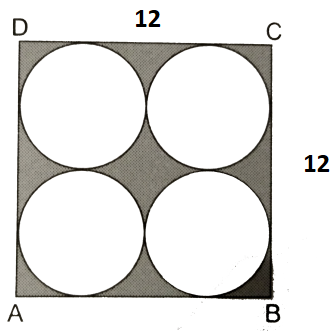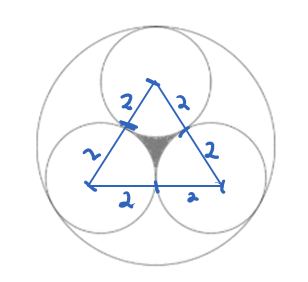(858) 436-7220

Request A FREE Consultation

# Mathematical Patterns and Where to Find Them

by | Sep 1, 2021

Written by: David Lord

Mathematical patterns exist all around us, but they can remain unseen by the untrained eye.

For example take this flower below:

Most people look at the sunflower radially and do not notice any specific pattern.  The seeds seem randomly placed, but there is a hidden pattern to it if we know where to look!  Try tracing out in a spiral, and you may be surprised by what you find!

Biologists have spent time counting the spirals and they found that they typically end in either 13, 21, 34, or 55.   However, a direct biological or physical cause has yet to be established.

These numbers are part of the fabulous Fibonacci sequence of 1, 1, 2, 3, 5, 8, 13, 21, 34, 55 and so forth.

Another natural example of hidden patterns was brought up by Elsa in the Frozen movie:

Fractals are never ending patterns that repeat with similar shapes with different scales.

The Koch Snowflake is a classic fractal that starts as an equilateral triangle.  Along each edge, another triangle is formed.  This continues indefinitely as you can below.

Koch Snowflake Fractal

Once you start noticing these patterns, you can start seeing them everywhere!   For example, students are often awestruck with the speed that I can solve some of the SAT questions.

Take the following question:

What is the area of the shaded region?My instincts recommend that I should find the area of the Whole Square and subtract the areas of the 4 circles, or 122 – 4*32*𝜋  This is a common skill on the test where you will either need to find the area directly or by combining the areas of common shapes.

My initial instincts tell me that I should be able to use the same approach but it is not clear which areas that we need to subtract. By looking at where the circles intersect, I can draw the radii of the circles.Now it is obvious that we have an equilateral triangle and we have 3 sectors that we have to subtract from.  I can proceed and show the derivation of the work, but there is clearly one answer that fits the pattern!

Since the area of the sector is a fraction of the whole circle, it means we should look for an answer choice that subtracts some value of 𝜋.   So the answer must be B

That saves a lot of work and time, but the crazy thing is that there is even a faster approach.

We know that one of the circles has an area of 4𝜋 or 12.57.  If you look at the answer choices, we can approximate them as

1. 3.46
2. 0.64
3. 5.64
4. 6.93

The area is less than an ¼ of the area of one circle so it must be B.

The point of this exercise is that while questions have a formulaic approach, I encourage my students to reexamine their work, and see if the solution makes sense.  If instead we had gotten D, I would know to double check my work, and look for a reason why I got an answer that is much bigger than expected.  Students who take this step and think critically about their answers, start identifying the many patterns on the SAT and ACT tests.

Thank you for joining me on this journey and I hope that you found this to be a prime source of information.

David Lord

Director of Math and Science, mathophile and all around nerd.

#